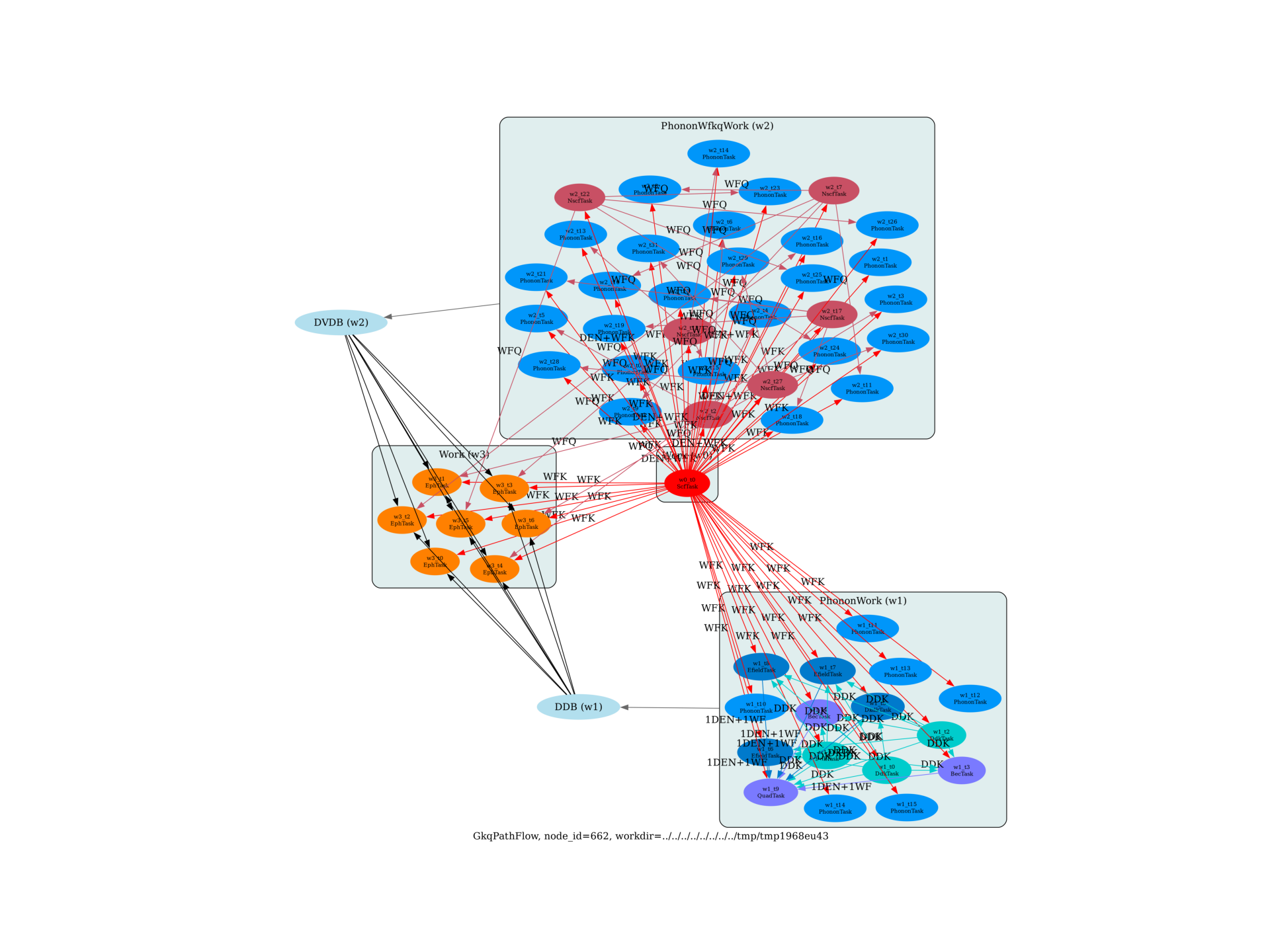Flow to compute e-ph matrix elements along a q-path¶

This example shows how to compute the e-ph matrix elements in AlAs along a q-path with AbiPy flows. The final results are stored in the GKQ.nc file (one file for q-point) in the outdata of each task.

import sys
import os
import abipy.abilab as abilab
import abipy.data as abidata

from abipy import flowtk

def make_scf_input(ngkpt):
"""
This function constructs the input file for the GS calculation:
"""
structure = abidata.structure_from_ucell("AlAs")
pseudos = abidata.pseudos("13al.981214.fhi", "33as.pspnc")
gs_inp = abilab.AbinitInput(structure, pseudos=pseudos)

gs_inp.set_vars(
nband=6,
ecut=6.0,
ngkpt=ngkpt,
nshiftk=1,
shiftk=[0, 0, 0],
tolvrs=1.0e-10,
)

return gs_inp

def build_flow(options):
"""
Create a `Flow` for the computation of e-ph matrix elements
"""
# Working directory (default is the name of the script with '.py' removed and "run_" replaced by "flow_")
if not options.workdir:
options.workdir = os.path.basename(sys.argv).replace(".py", "").replace("run_", "flow_")

# Use 2x2x2 both for k-mesh.
# Build input for GS calculation
scf_input = make_scf_input(ngkpt=(2, 2, 2))

# corresponding to a [2, 2, 2] q-mesh.
ngqpt = (2, 2, 2)

# Create flow to compute all the independent atomic perturbations
# Use ndivsm = 0 to pass an explicit list of q-points.
# If ndivsm > 0, qpath_list is interpreted as a list of boundaries for the q-path
qpath_list = [[0.0, 0.0, 0.0], [0.01, 0, 0], [0.1, 0, 0],
[0.24, 0, 0], [0.3, 0, 0], [0.45, 0, 0], [0.5, 0.0, 0.0]]

from abipy.flowtk.eph_flows import GkqPathFlow
flow = GkqPathFlow.from_scf_input(options.workdir, scf_input,
ngqpt, qpath_list, ndivsm=0, with_becs=True,
ddk_tolerance={"tolwfr": 1e-8})

return flow

# This block generates the thumbnails in the AbiPy gallery.
# You can safely REMOVE this part if you are using this script for production runs.
__name__ = None
import tempfile
options = flowtk.build_flow_main_parser().parse_args(["-w", tempfile.mkdtemp()])
build_flow(options).graphviz_imshow()

@flowtk.flow_main
def main(options):
"""
This is our main function that will be invoked by the script.
flow_main is a decorator implementing the command line interface.
Command line args are stored in `options`.
"""
return build_flow(options)

if __name__ == "__main__":
sys.exit(main())Run the script with:

run_gkq.py -s

then use:

abirun.py flow_phonons history

Total running time of the script: ( 0 minutes 2.809 seconds)

Gallery generated by Sphinx-Gallery